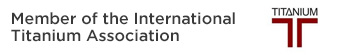## Dependable, Competitive, Customer Focused.

Building relationships in the metals industry since 1948.

# Weight Calculator

Estimate the total weight of your titanium order with our user-friendly calculator

## Please fill in the diameter, length, and quantity of your order.

Note : The dimensions must be entered as inches.

## Titanium Round Bars:

Diameter =
Length =
Quantity=
Total Weight (lbs) =
Weight Per Piece (lbs) =

## Titanium Rectangles and Plates:

Thickness=
Width=
Length =
Quantity=
Total Weight (lbs) =
Weight Per Piece (lbs) =

## Titanium Rings:

Outer Diameter =
Inner Diameter =
Length =
Quantity=
Total Weight (lbs) =
Weight Per Piece (lbs) =

 Pounds = Kilogram =

 Milimeters = Inches =

 MPA = KSI =

 Cel = Faren =

#### Disclaimer: Laube Titanium’s calculators estimate theoretical titanium weights. Actual weights may vary significantly depending on the material and tolerances.